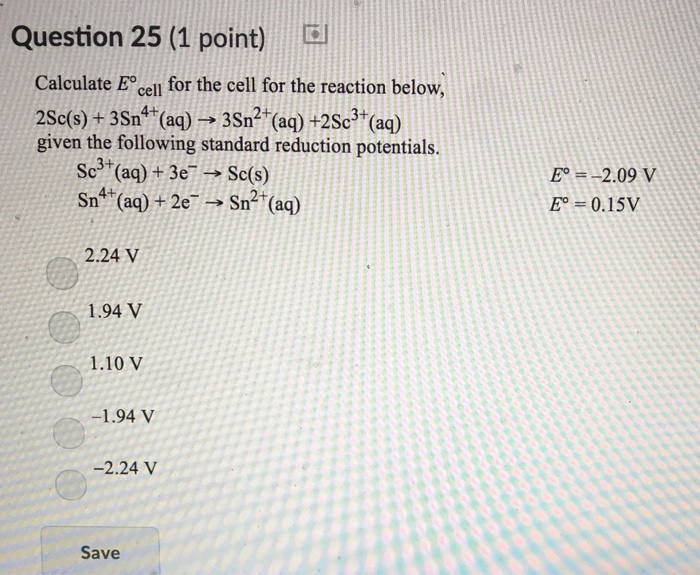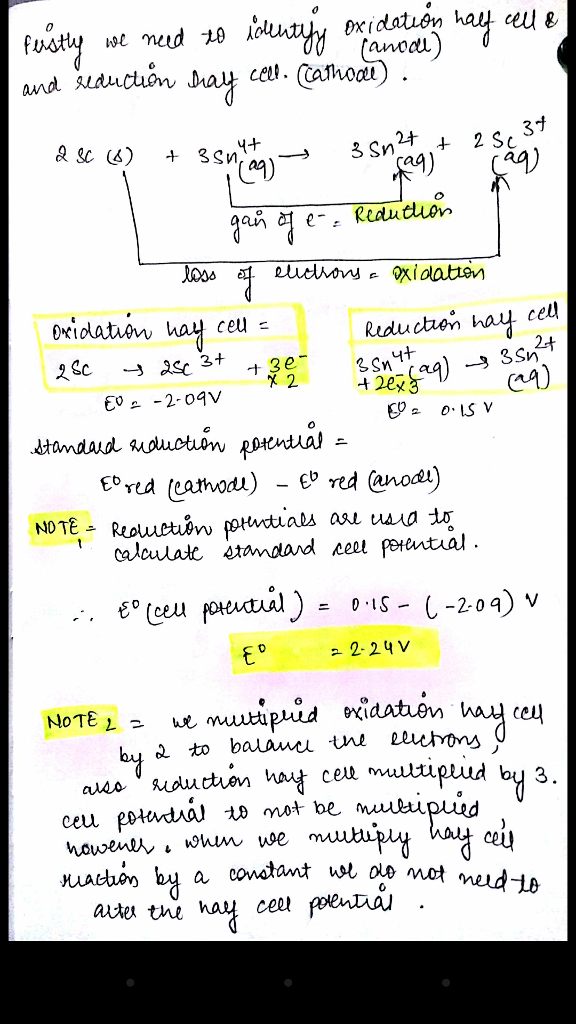# Question & Answer: Calculate E degree _cell for the cell for the reaction below, 2Sc(s) + 3Sn^4+(aq) rightarrow…..Calculate E degree _cell for the cell for the reaction below, 2Sc(s) + 3Sn^4+(aq) rightarrow 3Sn^2+(aq) + 2Sc^3+(aq) given the following standard reduction potentials. Sc^3+(aq) + 3e^- rightarrow Sc(s) E degree = -2.09 V Sn^4+(aq) + 2e^- rightarrow Sn^2+(aq) E degree = 0.15 V 2.24 V 1.94 V 1.10 V -1.94 V -2.24 V